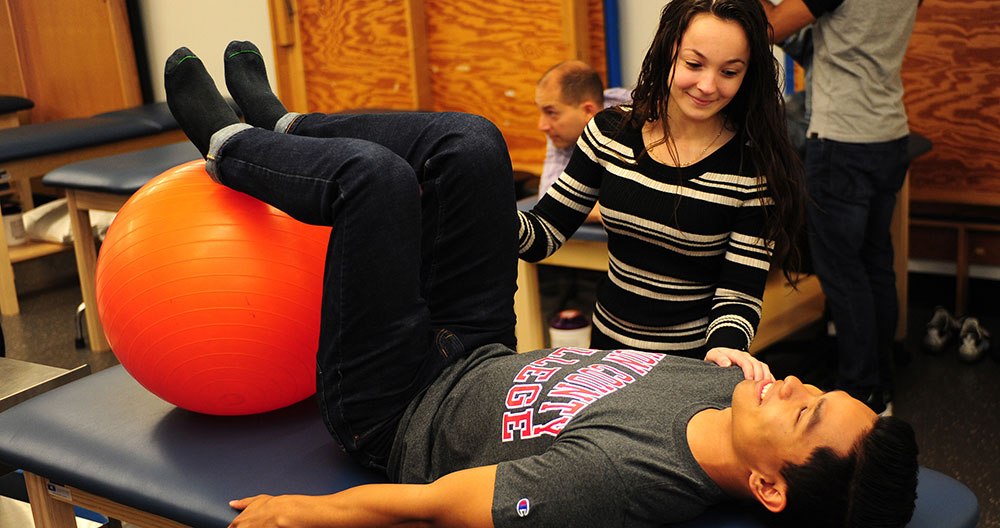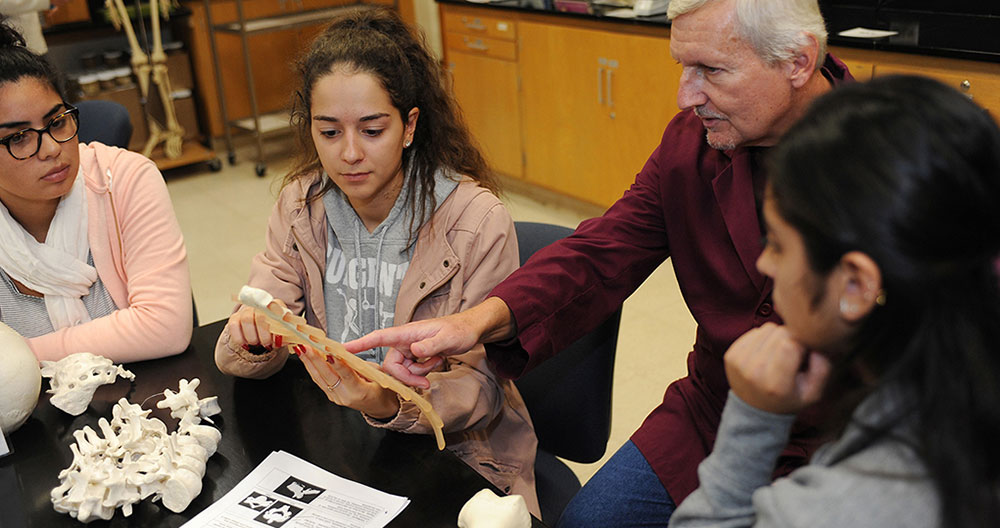Union County College

# Mathematics

Mathematics is both a science and an art. In our ever-changing world, mathematics is a constant at the root of analytical thinking. As the foundation for studies in science, technology, economics, and other disciplines, the demand for graduates with strong mathematical backgrounds is increasing. Whether to better grasp basic applications in our society, to come to understand the beauty and utility of mathematics in our natural world, to recognize its connections to the humanities, or to apply its intricate relationships in advanced technical fields, the study of mathematics develops one’s ability to think critically, reason logically and quantitatively, and appreciate the interconnectedness of the disciplines pragmatically.
The specific objectives of this program are that the graduate will be able to demonstrate:
• Demonstrate critical thinking, analytical reasoning, and problem solving skills
• Communicate mathematics effectively, applying its unique language and symbolic system
• Identify and apply mathematical theorems
• Write clear and logical discussions, supporting one’s point of view.
• Formulate and evaluate possible solutions to problems, and select and defend the chosen solutions with mathematical proofs or by scientific method as appropriate
• Translate quantifiable problems into mathematical terms and solve these mathematical models
• Interpret charts & graphs, and draw appropriate conclusions
• Demonstrate an awareness of mathematical principles and applications in an ever-changing society
• Demonstrate comprehension of mathematical concepts and algorithms through applications, making real-world connections in order to keep pace with contemporary progress in a variety of fields
• Use technology in analyzing and solving mathematical problems
• Solve mathematical problems independently and cooperatively as part of a team
• Comprehend and evaluate resources
• Demonstrate comprehension of mathematical concepts through the ability to teach them effectively to others
• Discuss the fact that all the disciplines have been affected by mathematical developments
• Demonstrate knowledge of important historical figures across cultures who have contributed to the development of mathematics
• Recognize the need for continuing efforts to assure the study and applications of mathematics in our society